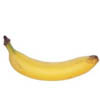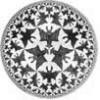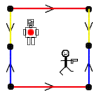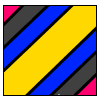Search by Topic

Resources tagged with Non Euclidean Geometry similar to Flight Path:

Filter by: Content type:
Age range:
Challenge level:

There are 10 results

Broad Topics > 3D Geometry, Shape and Space > Non Euclidean GeometryFlight Path

Age 16 to 18 Challenge Level:

Use simple trigonometry to calculate the distance along the flight path from London to Sydney.Curvature of Surfaces

Age 16 to 18

How do we measure curvature? Find out about curvature on soccer and rugby balls and on surfaces of negative curvature like banana skins.How Many Geometries Are There?

Age 16 to 18

An account of how axioms underpin geometry and how by changing one axiom we get an entirely different geometry.Spherical Triangles on Very Big Spheres

Age 16 to 18 Challenge Level:

Shows that Pythagoras for Spherical Triangles reduces to Pythagoras's Theorem in the plane when the triangles are small relative to the radius of the sphere.Weird Universes

Age 16 to 18 Challenge Level:

Consider these weird universes and ways in which the stick man can shoot the robot in the back.Over the Pole

Age 16 to 18 Challenge Level:

Two places are diametrically opposite each other on the same line of latitude. Compare the distances between them travelling along the line of latitude and travelling over the nearest pole.Geometry and Gravity 1

Age 11 to 18

This article (the first of two) contains ideas for investigations. Space-time, the curvature of space and topology are introduced with some fascinating problems to explore.Pythagoras on a Sphere

Age 16 to 18 Challenge Level:

Prove Pythagoras' Theorem for right-angled spherical triangles.When the Angles of a Triangle Don't Add up to 180 Degrees

Age 14 to 18

This article outlines the underlying axioms of spherical geometry giving a simple proof that the sum of the angles of a triangle on the surface of a unit sphere is equal to pi plus the area of the. . . .Torus Patterns

Age 16 to 18 Challenge Level:

How many different colours would be needed to colour these different patterns on a torus?